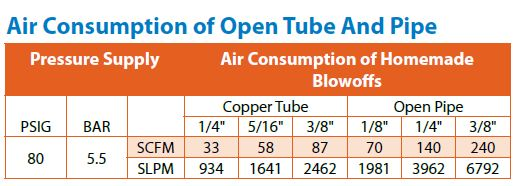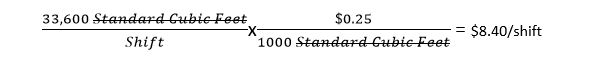## How Do I Estimate The Cost Of My Compressed Air?

One of the best features of EXAIR products is the engineering behind the designs.  For example, our nozzles are designed to generate a maximum force possible per CFM of compressed air.  This means that the compressed air consumed by the device is at its maximum possible efficiency, which in turn reduces the compressed air demand in an application, reducing the cost of the solution.

But, how do you determine the cost of a compressed air driven product?

Step 1 – Quantify flow

The first step to determine compressed air cost is to quantify the flow rate of the product.  Most pneumatic equipment will have a spec sheet which you can reference to determine air consumption, but open pipe blowoffs and drilled holes won’t provide this type of information.  In those cases, or in any case where the compressed air flow is unknown or questionable, a compressed air flow meter can be used.  (We have Digital Flowmeters for use on compressed air piping, with or without data logging capability, and with serial or wireless communication.)

Step 2 – Calculate flow over time

Once the flow rate is known, it’s time to determine flow rates per day/week/month/year.  To do so, we will perform a bit of short and easy math.  What we will do, is use the known flow rate of the device, and multiply this by the total time in operation to determine daily, weekly, monthly, and annual usage rates.  For example:A 1/8” open pipe blowoff will consume 70 SCFM.  In an 8 hour shift there are 480 minutes, resulting in a total consumption of 33,600 SCFM per 8 hour shift.Step 3 – Determine cost

With a quantified flow rate, we can now determine the cost.  Many facilities will know the cost of their compressed air per CFM, but for those which don’t, a cost of (\$0.25/1000 standard cubic feet) can be used.  This value is then multiplied by the total compressed air consumption from above, to give a quantified dollar amount to the compressed air driven device.

Using the flow rate from above:If (1) shift is run per day, 5 days per week and 52 weeks per year, this open pipe blowoff will have an annual cost of \$2,184.00.

Step 4 – Compare

At this point we know the real cost of the device.  The benefit to quantifying these flow rates, is when making a comparison to an alternative such as an engineered solution.  For example, if we were to replace the open pipe blowoff reference above with an EXAIR 1010SS 1/8” NPT nozzle, the compressed air demand would drop to 13 SCFM, yielding the following flow rates and costs:If (1) shift is run per day, 5 days per week and 52 weeks per year, this open pipe blowoff will have an annual cost of \$405.60.

Comparing these two solutions on an annual basis yields a difference of \$1,778.40.  This means an air savings which correlates to \$1,778.40 per year – just by replacing ONE open pipe blowoff with an engineered solution.  Replacing multiple open pipe blowoffs will yield repeat savings.